# Data Analysis

### Course Description

• This course is sign on statistics.
• It will told us some models, and explain them station.
• like regression, ANOVA, ANCOVA, and so on

### key point before test

1、作业1的第1题，第1-2章的课件。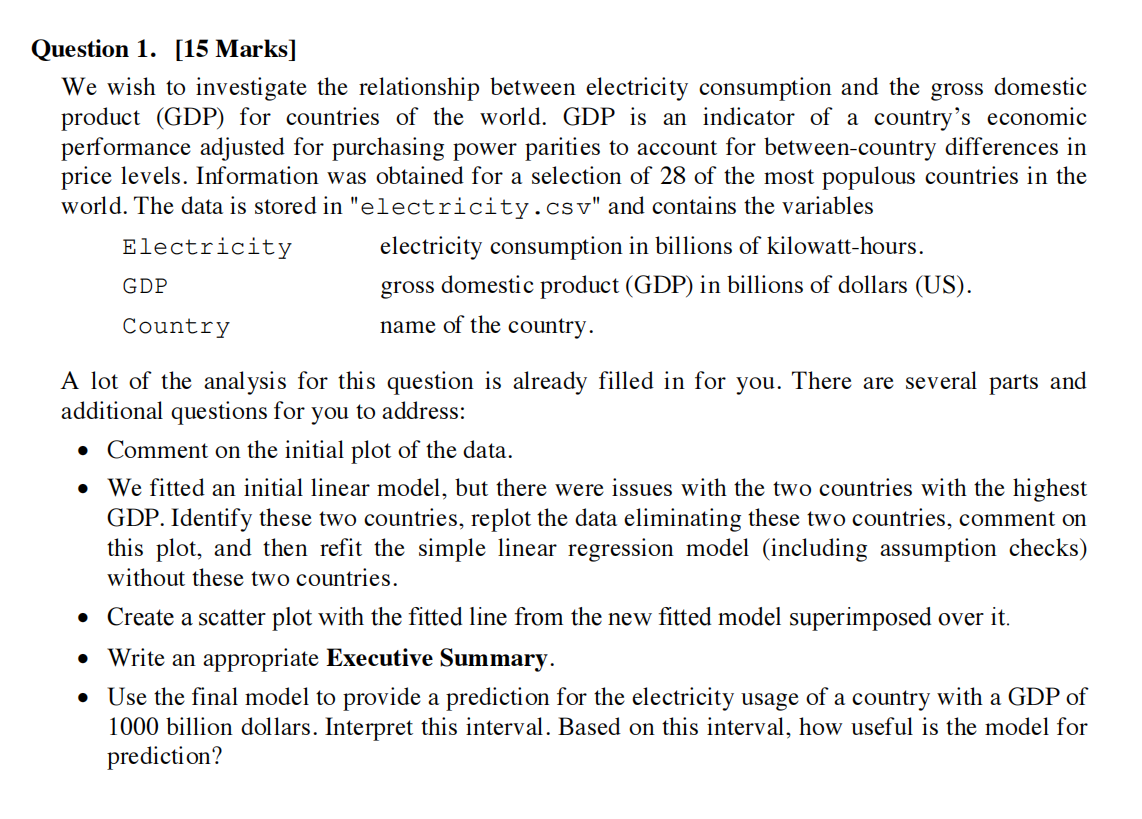• Look at the question：

• Goal: Inverstigate the relationship between electricity consumption and GDP.

• Data: A file named electricity.csv, coverd Electricity, GDP, Country name

• T1: Comment the initial plot of the data

• word notes:

• If we want to comment the initial plot of the data, we need get this plot before.

``````elec.df <- read.csv("electricity.csv")
plot(Electricity~GDP, data=elec.df)
# show the data plot, and entitled it
``````
• and the outcome is here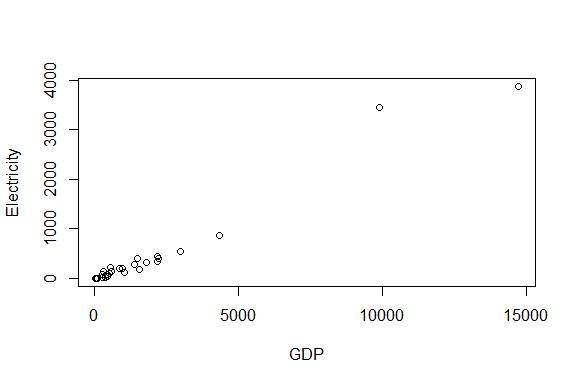In the plot, when GDP less than 5000, It is clearly that there are some relationship between GDP and Electricity.

the Electricity will increased by growth with GDP.

• T2: We fitted an initial linear model, but there were issues with the two countries with the highest GDP. Identify these two countries, replot the data eliminating these two countries, comment on this plot, and then refit the simple linear regression model (including assumption checks) without these two countries.

• word notes:

• First, calculate the residuals and fitted valued.

``````elecfit1=lm(Electricity~GDP,data=elec.df)
# function lm: calculate the residuals and fitted value.
plot(elecfit1,which=1)
# show the plot
word notes:{calculate:计算, residuals:残差, fitted value: 拟合值}
``````
• The outcome is here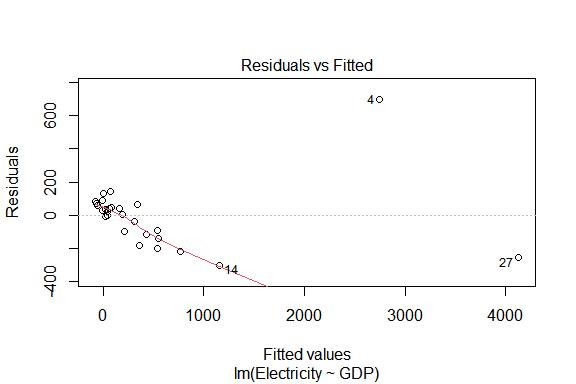• Then, get the norm check to this data

``````normcheck(elecfit1)
``````
• The outcome in here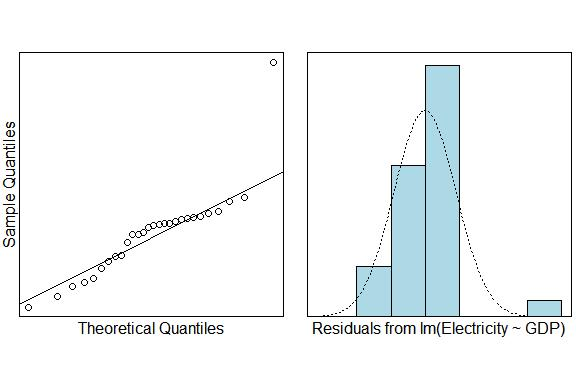• Then, get the Cook`s distance plot

``````cooks20x(elecfit1)
``````
• The outcome in here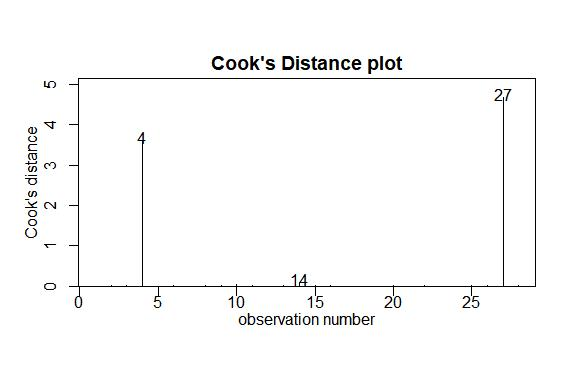• In the cooks distance plot, there are two data is far from others. Its the 4th and 27th. It have a disproportionate impact to the model. And, according to residuals and fitted valued, we can infer that those two countries is which GDP more than 5000.

• now, del those data and replot data.

``````data=elec.df[elec.df\$GDP<5000,]
# del data which GDP over 5000
plot(Electricity~GDP,data)
# and show the plot
``````
• Okay, now we only have the last part: check again

``````elecfit2=lm(Electricity~GDP,data=elec.df[elec.df\$GDP<6000,])
plot(elecfit2,which=1)
# get data, and show original plot

normcheck(elecfit2)
# norm check

cooks20x(elecfit2)
# cooks distance
``````
• T3 Create a scatter plot with the fitted line from the new fitted model superimposed over it.

• word notes:

``````plot(Electricity~GDP,data=elec.df[elec.df\$GDP<6000,])
# get plot
elecgdp.fit=lm(Electricity~GDP,data=elec.df[elec.df\$GDP<6000,])
abline(elecgdp.fit,lty=2,col="red")
``````
• T4 Write an appropriate Executive Summary.

• word notes:
• We wish to investigate the relationship between electricity consumption and the gross domestic product (GDP) for countries of the world. First,we read in and inspect the data and get an initial plot. Then,we use lm() to fit a appropriate linear model and use normcheck() and cooks20x() to do model checks. To make estimation credible,we eliminate two countries with GDP greater than 6000. Because their Cook’s distances are greater than 0.4. Then,we replot data and fit a more appropriate linear model by same way. Finally,we create a scatter plot with the fitted line from your model superimposed over it.
• word notes:
• We want to find a relationship between the GDP and the electricity consumption. First, we read the data from file and get an initial plot. And then, we use the function lm to fit a appropriate linear model and use two function named normcheck and cooks20x to do model checks. We find two countries have different data, because the cooks distance is more than 0.4. So, we eliminate them, and get initial plot again, and do model check again. Finally, we create a scatter plot with the fitted line from model superimposed over it.

2、会对x-y的散点图进行评论。（课件第1章第20页）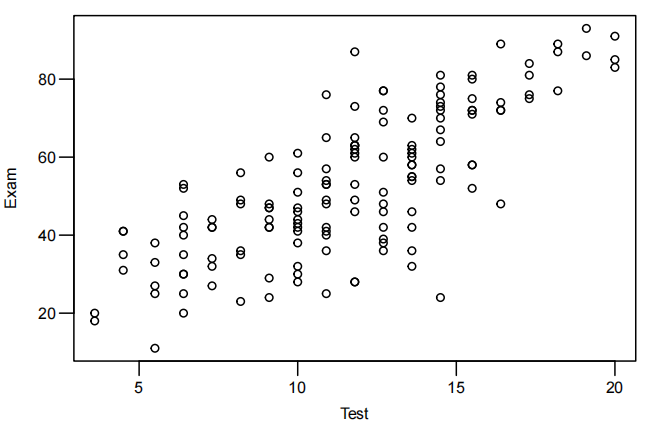• Step one: some fixed describtion

Looking at this plot, it is clear that there is some relationship.

but there is also a lot of variability in exam score amongst students with the same test score, especially in the middle of the data.

3、会对同一数据应用不同模型进行拟合的图进行评论。（课件第2章第,31页）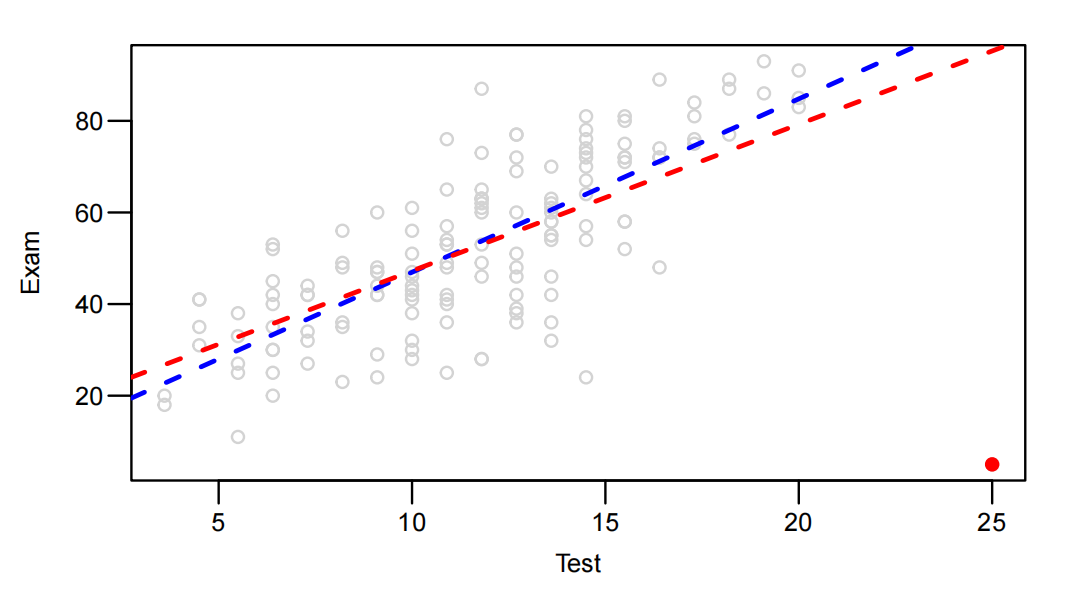• First, judge the type of two models(linear), describe the positive/negative, the slope of them.

• Next, Prediction results: When is it close, when is it far away, and why? As can be seen in the figure, the two lines are close to each other in which range between x, and far away in which range?.

• The reasons for this phenomenon are; the existence of points; The middle block has more data, while the two sides have less data. When the amount of data is large, different models have different resistance to outliers

• Like:

• They are two positive linear model, at first, the scope of red one is more than the blue one, and then end, the station is opposite.
• Those two line is closed at middle, and be far at two side. I guess the reason is, the point almost focus on the middle, only few on the side.

4、知道summary这个函数输出结果中每个数字的含义。（课件第2章第40-46页）

• This part only about translate.
• word notes:
• Estimate 估计值
• Std. 标准差
• Error 误差
• Intercept 截距
• Slope 斜率
• Residual standard error 残差标准误差
•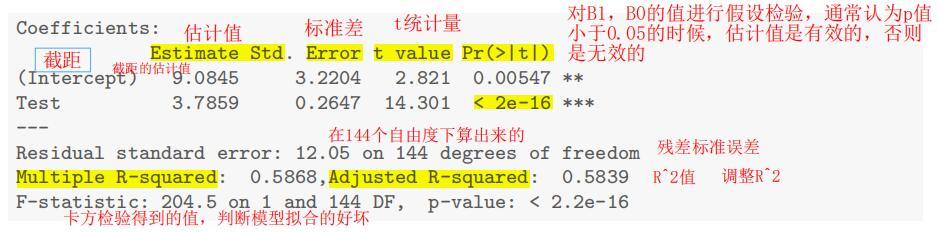5、会结合程序描述E(y)的置信区间（CI）和y的预测区间（PI）的具体含义。（课件第2章第48-50页）

6、Methods and Assumption Checks的写法。（参考作业1的第1题）

1、第6章课件，第6章案例。
2、应用乘法模型对数据拟合的三个原则。（课堂笔记L06-1）
3、乘法模型的形式。（第6章案例）
4、Executive Summary的写法。（第6章案例）

## Data Analysis

Course DescriptionThis course is sign on statistics.It will told us some models, and explain them station.like regression, ANOVA, ANCOVA, and so onkey…